A central angle of a circle has an angle measure of 1° if it subtends an arc that is 1/360 of the circumference of the circle. This form of angle measure is quite common. Another form of angle measure that is in use is radian measure. If a central angle subtends an arc that is equal to the radius of the circle (Figure ), then the central angle has a measure of one radian.Figure 1

If a central angle θ of a circle with radius r subtends an arc of length q (Figure 1 ), then its radian measure is defined as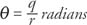Because both q and r are in the same units, when q is divided by r in the preceding formula, the units cancel. Therefore, radian measure is unitless.

Example 1: What is the radian measure of a central angle in a circle with radius 6 m if it subtends an arc of 24 m?(Note that if no units are listed for an angle measure, it is assumed to be in radians.)

If θ is one complete revolution, then the subtended arc is the circumference of the circle. In this case,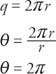Because one complete revolution is 360°,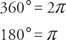The fact that 180° is the same as π radians is extremely important. From this relationship, the following proportion can be used to convert between radian measure and degree measure:Example 2: What is the degree measure of 2.4 rad?Example 3: What is the radian measure of 63°?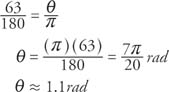The radian measures of many special angles follow directly from the radian‐degree relationships. Some of these are summarized in Table 1 .The areas of sectors of a circle are directly proportional to the measures of their central angles and directly proportional to the arcs subtended by the central angles (Figure  2 ).Figure 2
Sector area.Example 4: Find r given that α = 14π and θ = π/2.Example 5: Find θ if A = 6 and r = 4.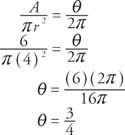Example 6: What is the angle measure, in radians, of the acute angle formed by the minute and hour hands of a clock at 7:15?

The hour hand moves 1/12 of a complete revolution each hour. Therefore, every 15 minutes (one quarter of an hour), the hour hand moves 1/48 of a complete revolution. Therefore, at 7:15, the hour and minute hands are 17/48 of a revolution apart.Example 7: Find the area of the shaded portion of the sector of the circle shown in Figure  7.Figure 3
Drawing for Example 7.

First, use the Pythagorean theorem to find the value of a.The area of the triangular (unshaded) portion of the sector can be calculated using the area formula of a triangle.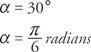It follows thatTherefore,

area of shaded portion = area of total section − area of unshaded portion

area of shaded portion =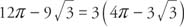area of shaded portion ≈ 22.11.